# A charge of -7.4 µC is traveling at a speed of 8.6 106 m/s in a...

A charge of -7.4 µC is traveling at a speed of 8.6 106 m/s in a region of space where there is a magnetic field. The angle between the velocity of the charge and the field is 52°. A force of magnitude 4.7 10-3 N acts on the charge. What is the magnitude of the magnetic field?

Force on the electron in the magnetic field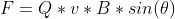Q - charge =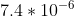v - velocity =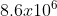F- Force =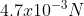B- Mgnetic Filed = ??

Theta =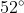So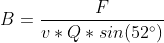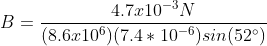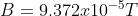#### Earn Coin

Coins can be redeemed for fabulous gifts.

Similar Homework Help Questions
• ### A charge of -6.09 μC is traveling at a speed of 6.65 × 106 m/s in...

A charge of -6.09 μC is traveling at a speed of 6.65 × 106 m/s in a region of space where there is a magnetic field. The angle between the velocity of the charge and the field is 53.4o. A force of magnitude 8.59 × 10-3 N acts on the charge. What is the magnitude of the magnetic field?

• ### A charge of -5.92 μC is traveling at a speed of 5.16 × 106 m/s in...

A charge of -5.92 μC is traveling at a speed of 5.16 × 106 m/s in a region of space where there is a magnetic field. The angle between the velocity of the charge and the field is 57.4o. A force of magnitude6.76 × 10-3 N acts on the charge. What is the magnitude of the magnetic field?

• ### A charge of -8.81 μC is traveling at a speed of 5.03 × 106 m/s in...

A charge of -8.81 μC is traveling at a speed of 5.03 × 106 m/s in a region of space where there is a magnetic field. The angle between the velocity of the charge and the field is 51.1o. A force of magnitude6.47 × 10-3 N acts on the charge. What is the magnitude of the magnetic field?

• ### A charge of -5.16 μC is traveling at a speed of 5.36 × 106 m/s in...

A charge of -5.16 μC is traveling at a speed of 5.36 × 106 m/s in a region of space where there is a magnetic field. The angle between the velocity of the charge and the field is 53.3o. A force of magnitude8.99 × 10-3 N acts on the charge. What is the magnitude of the magnetic field?

• ### Problem with question 2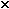A charge of -7.5 µC istraveling at a speed of 7.4 106 m/s in a region of spacewhere thereis a magnetic field. The angle between the velocity of the chargeand the field is 56°. A force ofmagnitude 4.0 10-3 N acts on the charge. What is themagnitude of the magnetic field?The answer I got for the problem was 86.93 but it marks it aswrong, can anyone help please?

• ### Physics 122 Test 4 Monday, Dec 4, 2107 Name Anthong Osod Answer ALL questions Show detailed...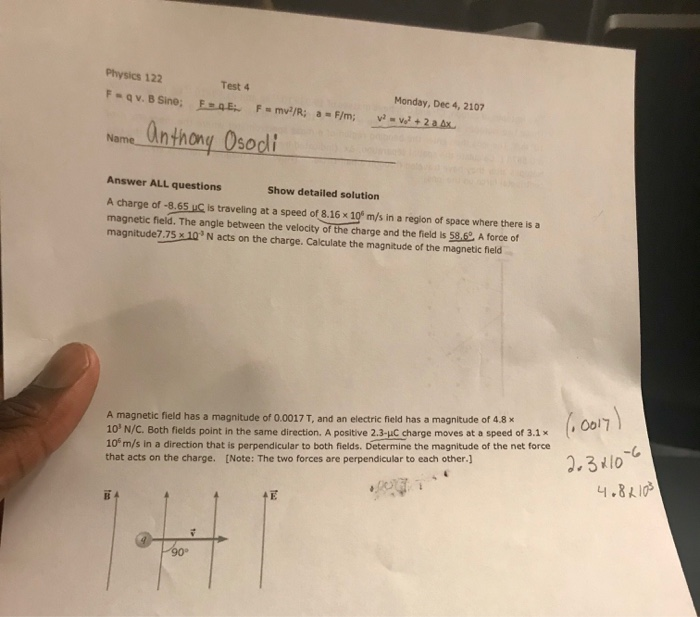Physics 122 Test 4 Monday, Dec 4, 2107 Name Anthong Osod Answer ALL questions Show detailed solution A charge of -8.65 uC Is traveling at a speed of 8.16x 10° m/s in a region of space where there is a magnetic field. The angle between the velocity of the charge and the field is 58.6 A forcef magnitude7.75 x 10 N acts on the charge. Calculate the magnitude of the magnetic field A magnetic field has a magnitude of 0.0017...

• ### An alpha particle (charge of +2e) moving with a speed of 5.5E6 m/s enters a region...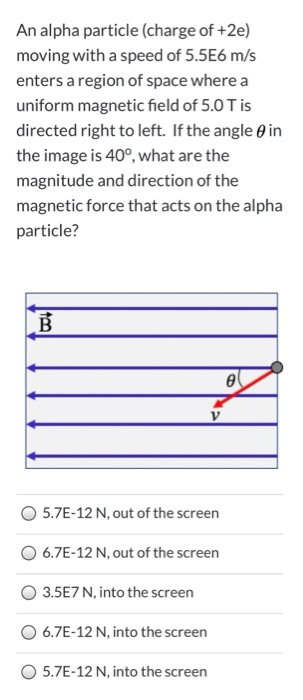An alpha particle (charge of +2e) moving with a speed of 5.5E6 m/s enters a region of space where a uniform magnetic field of 5.0 T is directed right to left. If the angle in the image is 40°, what are the magnitude and direction of the magnetic force that acts on the alpha particle? B e V 5.7E-12 N, out of the screen 6.7E-12 N, out of the screen 3.5E7 N, into the screen 6.7E-12 N, into the screen...

• ### (a) A proton, traveling with a velocity of 3.5 106 m/s due east, experiences a magnetic...

(a) A proton, traveling with a velocity of 3.5 106 m/s due east, experiences a magnetic force that has a maximum magnitude of 7.1 10-14 N and a direction of due south. What are the magnitude and direction of the magnetic field causing the force? magnitude     T (b) Repeat part (a) assuming the proton is replaced by an electron. magnitude T

• ### The speed of particle with 9e C charge is 7.6x104 m/s, and the magnitude of the...

The speed of particle with 9e C charge is 7.6x104 m/s, and the magnitude of the magnetic force is 15.4 x10-15 N. What is the magnitude of the magnetic field if the particle is traveling 16º to the field? (Recall e is the magnitude of the charge of an electron.)

• ### will rate, thanks! A proton travels with a speed of 5.05 106 m/s at an angle...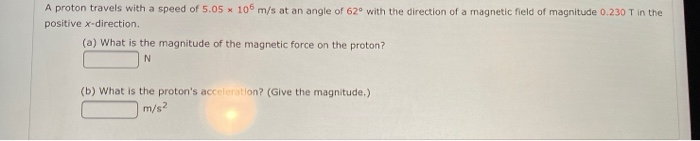will rate, thanks! A proton travels with a speed of 5.05 106 m/s at an angle of 62° with the direction of a magnetic field of magnitude 0.230 T in the positive x-direction. (a) What is the magnitude of the magnetic force on the proton? N (b) What is the proton's acceleration? (Give the magnitude.) m/s2